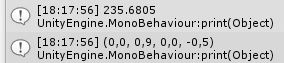# Quaternion.Slerp is not working as Quaternion.Euler has weird values

I just want a simple Rotation in the Y Axis with random values and have following code:

``````private void Rotate()
{
rotation = Random.Range(-360.0f, 360.0f);
print(rotation);
Quaternion qRotation = Quaternion.Euler(0, rotation, 0);
print(qRotation);
transform.rotation = Quaternion.Slerp(transform.rotation, qRotation, Time.deltaTime * rotationSpeed);
}
``````

But on Runtime the rotation is not working and the values for the Quaternion is also weird…Even when i put fix flaots into the Quaternion.Euler, the rotation is not happening as the values for the qRotation will alway between 0 and 1…

The qRotation value is correct. Quaternions do not show the rotation angle. Your problem is with how you are using the Slerp. That would need to be called each frame until it is complete. It interpolates from rotation A to rotation B by parameter T. Either set your rotation and then call the slerp every frame or use a coroutine like so;

``````public void RotateObj(float duration)
{
StartCoroutine(RotateActual(duration));
}

private IEnumerator RotateActual(float duration)
{
var startRot = transform.rotation;
var randomRot = Random.Range(-360f, 360f);
var endRot = Quaternion.Euler(0, randomRot, 0);

var t = 0f;

while (t <= 1f)
{
transform.rotation = Quaternion.Slerp(startRot, endRot, t);
t += Time.deltaTime / duration;
yield return null;
}

transform.rotation = endRot;
}
``````